【一猫汽车网】上汽通用凯迪拉克跃然推出了新一代旗舰车型凯迪拉克CT6车型，来源于底特律纯正血统的豪华汽车品牌凯迪拉克，一直在精心打造高品质的车型

【一猫汽车网 新车到店】经受住了2015年车市的寒冬，也经历了上海2016年的零下七八度的大寒冬，上汽通用凯迪拉克跃然推出了新一代旗舰车型凯迪拉克CT6车型，来源于底特律纯正血统的豪华汽车品牌凯迪拉克，一直在精心打造高品质的车型，如今，新一代的旗舰车型CT6于1月27日正式上市，售价区间在43.99万元-81.88万元，在第一时间，我们约到了凯迪拉克上海百联沪通汽车销售有限公司，下面我们一起来看看这款旗舰车型。实拍车型为顶配版的凯迪拉克CT6 40T 铂金版。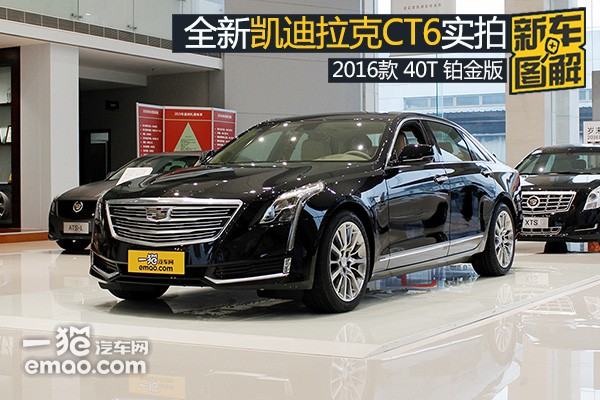拥有百年历史的凯迪拉克，在汽车界一直享有很高的荣誉，视为汽车生产的标准。而此次将要上市的凯迪拉克CT6是在上海金山桥生产基地量产车型，上海金山桥凯迪拉克生产基地拥有高度自动化的生产线，每年计划年产量16万台。凯迪拉克CT6车型定为在D级豪华轿车，运用了凯迪拉克众多黑科技，该车采用了全新的Omega后驱平台打造，因此拥有轻量化的全铝合金车身，顶配车型搭载了3.0L V6双涡轮增压发动机和8速手自一体变速箱。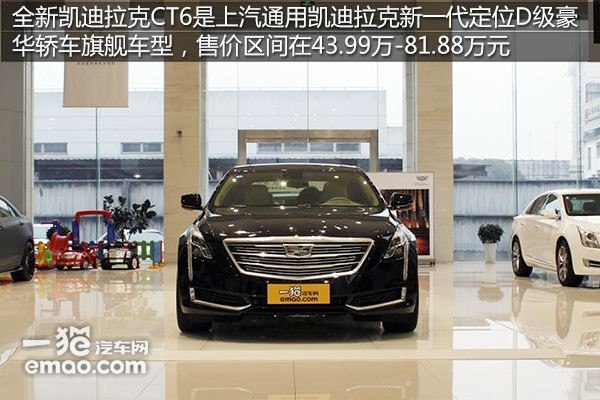据了解该车的定位官方并没有给出具体的竞争车型，但是从价格定为上可以大胆猜测，这款车的出现将有力挑战奥迪A8L、宝马7系和奔驰的S级。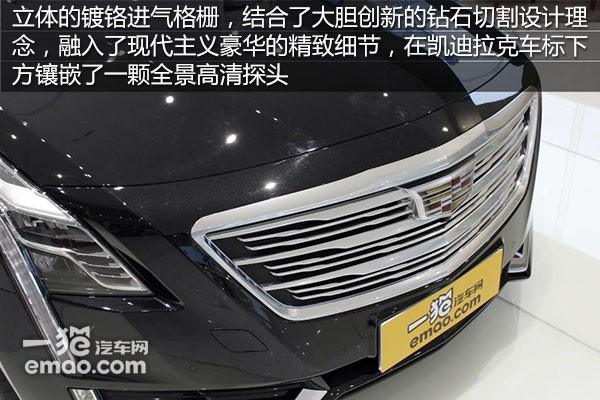外观上最醒目的是立体感十分强烈的镀铬进气格栅，凯迪拉克车标的下方镶嵌了一颗前视摄像头，全车完美镶嵌了208颗LED光源，大胆的钻石切割设计理念融入了现代主义豪华的精致细节。尺寸上，CT6的长宽高分别是5179mm、1879mm、1500mm，轴距为3109mm，基本达到了D级豪华轿车的水平。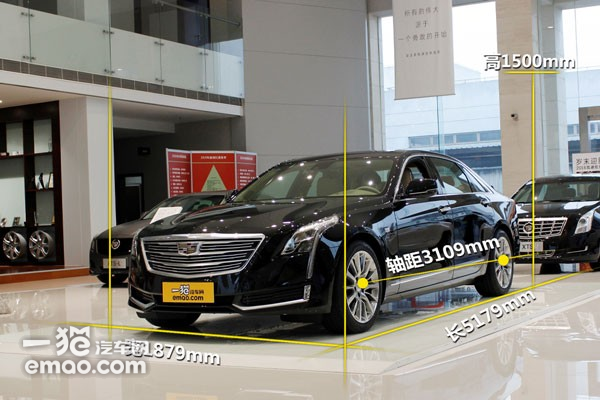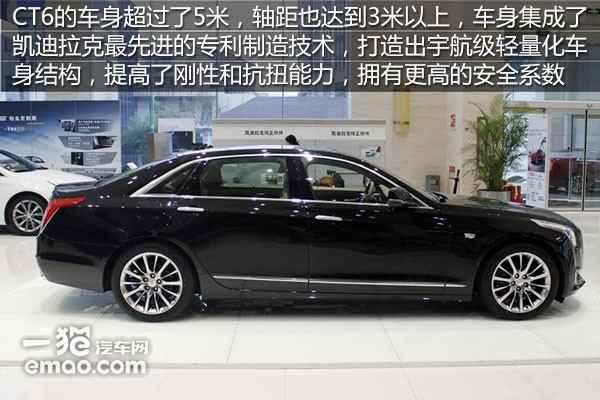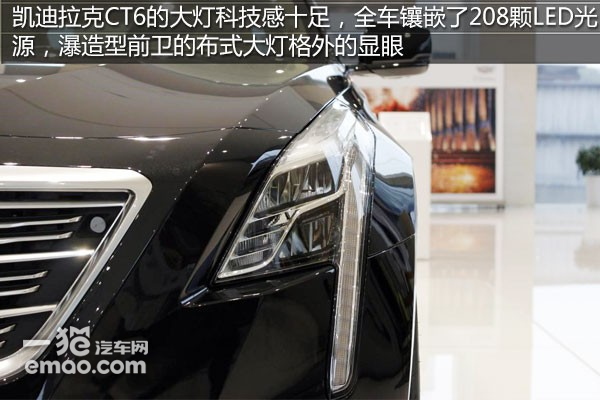凯迪拉克CT6采用的是设计前卫的星瀑式自适应非直射型LED前大灯，科技感和时尚感十足，在玩灯高手的奥迪面前也豪不示弱。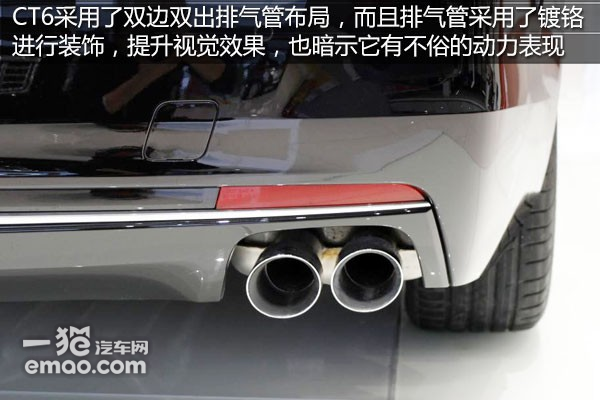凯迪拉克CT6整体车身设计线条流畅，虽然显得棱角分明，但是细节之处有限的十分的圆润细致。L型的尾灯细节却有着优美的弧线，单侧尾灯是由43个LED光源组成的。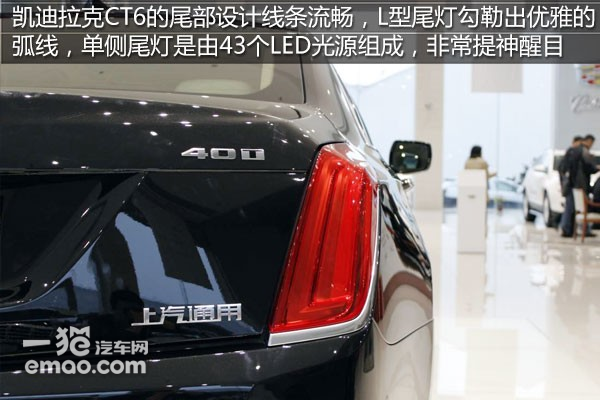内饰方面，凯迪拉克用料十分考究，将昂贵的各种实木和顶级的Opus牛皮以及金属碳纤维饰板精心搭配在一起，采用了法式双缝线工艺，呈现出尊贵奢华的乘坐体验。这也是美系豪华车一贯的强项做法。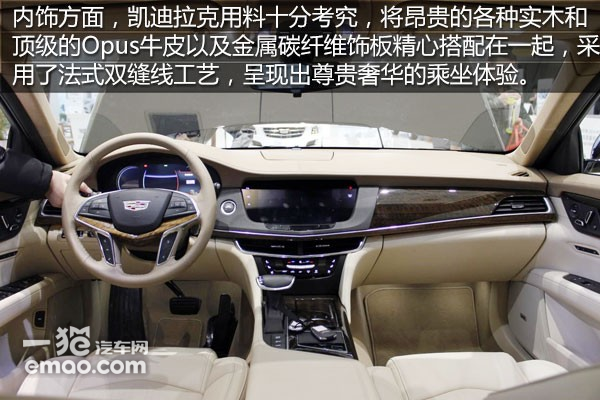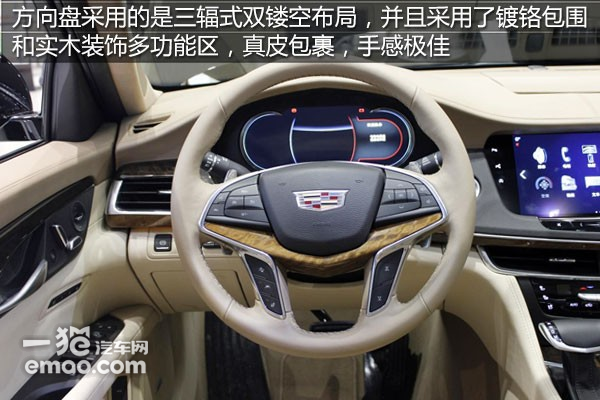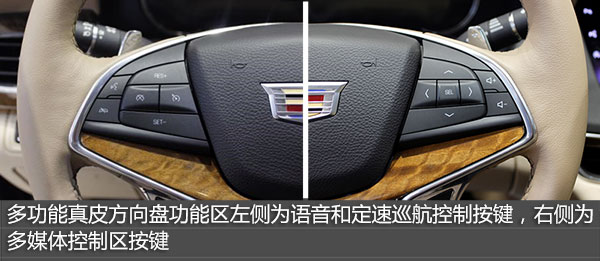全新凯迪拉克CT6搭载的全新升级的12寸液晶数字式仪表盘科技感很高，分辨率显示很清晰，显示的内容丰富，集车速、胎压检测、发动机转速、水温和燃油于一体，还能够切换成多种主题。另外该车也配备了抬头显示功能，能够便捷的将实时车况信息反馈给驾驶者。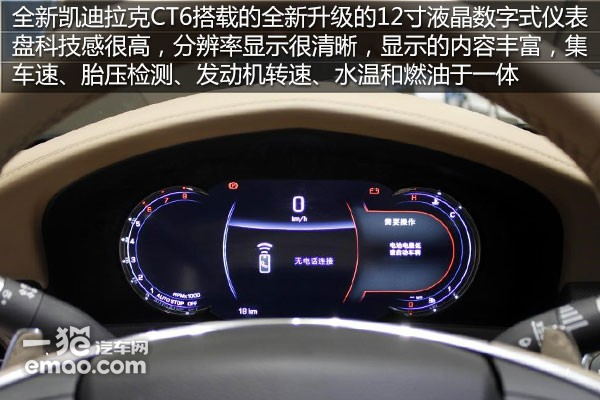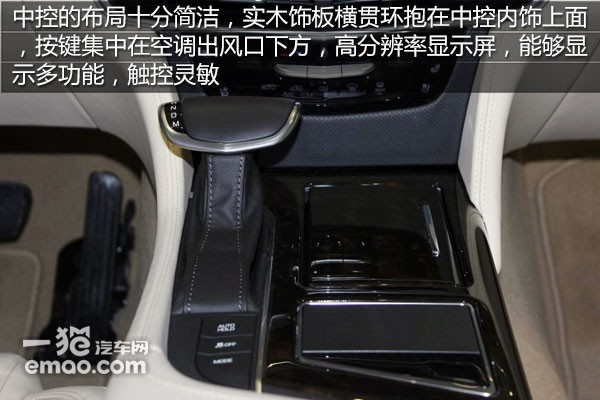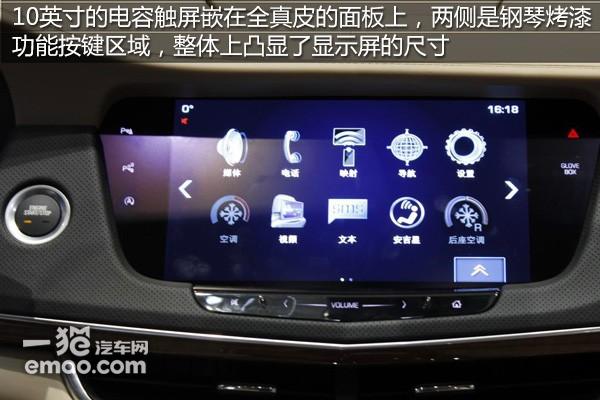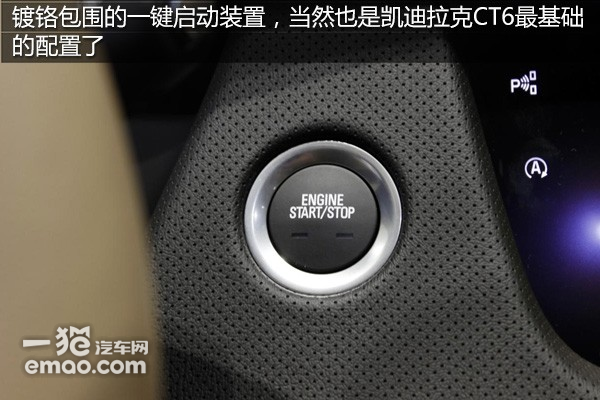中央扶手上的全掌触摸控制板支持手写输入，反应速度上体验度还是很不错的，杯架的布局更方便副驾驶乘客的使用，自动驻车按键和ESP开关按键以及行驶模式选择按键都按顺序分布在中央扶手区。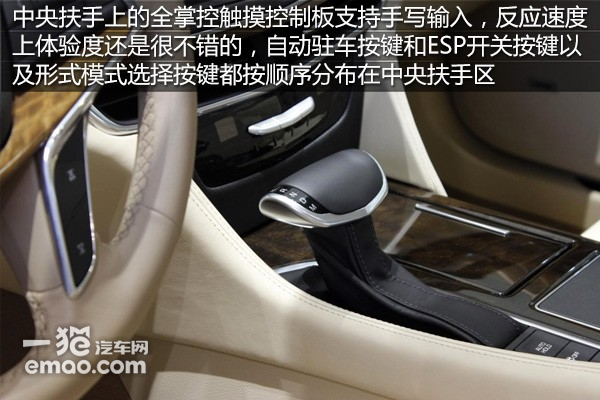凯迪拉克CT6座椅选用的是采用率只有四分之一的顶级Opus真皮，另外使用Cut-and-Sewn法式双缝线工艺缝制，前排座椅还集成了通风、按摩和加热的功能。后排座椅为14向电动调节可倾斜行政座椅，用料做工和前排一致，享受零压力的乘坐体验。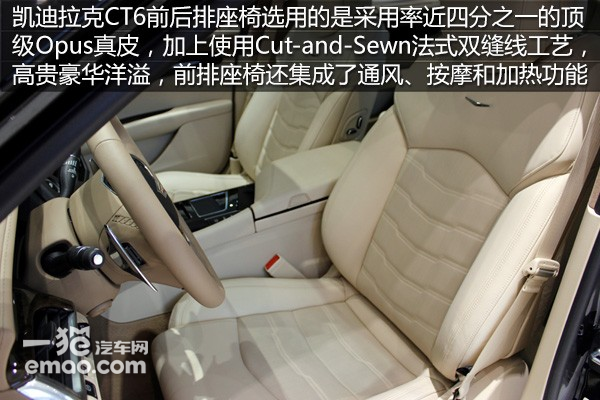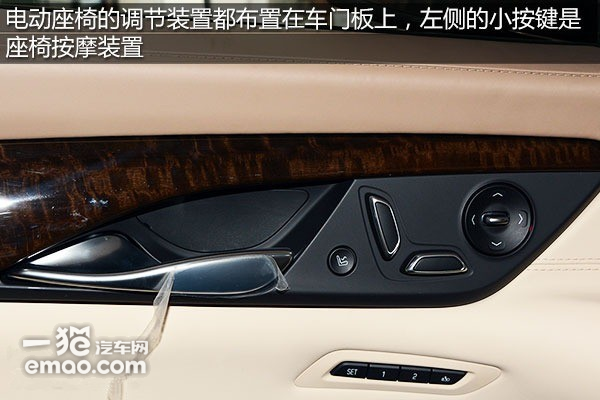空间方面，前排空间的体验空间非常的充裕，加上前排拥有座椅按摩功能，驾驶会有很不错的享受乐趣。前排的座椅柔软，头枕支撑到位，膝部接触全部是真皮包裹，头部空间很宽裕。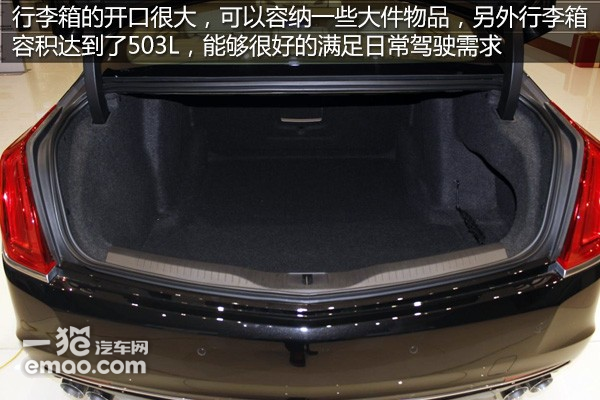动力方面，凯迪拉克为CT6配备了两款动力总成，分别是代号LTG的2.0T直列四缸的涡轮增压发动机和代号LGW的3.0L V6涡轮增压发动机，2.0T的最大功率203KW(276PS)，最大输出扭矩400N·m/3000-4300rpm;3.0T的最大功率298KW(405PS)，最大输入扭矩543N·m/2500-5000rpm，与之相匹配的传动系统是通用旗下最新的8速手自一体变速箱。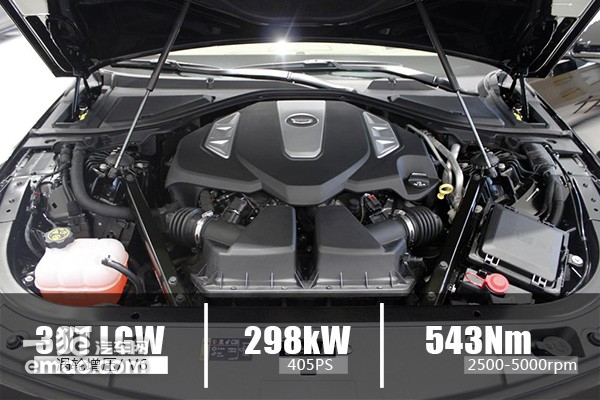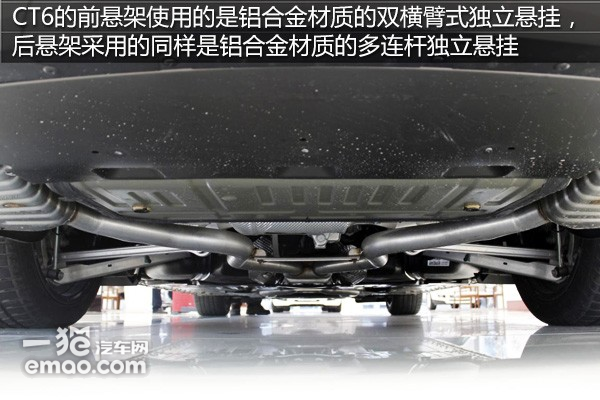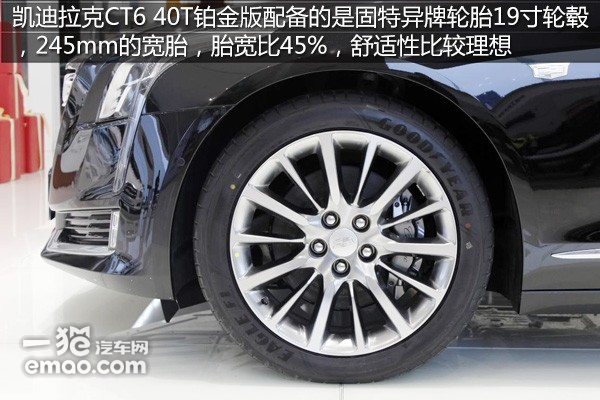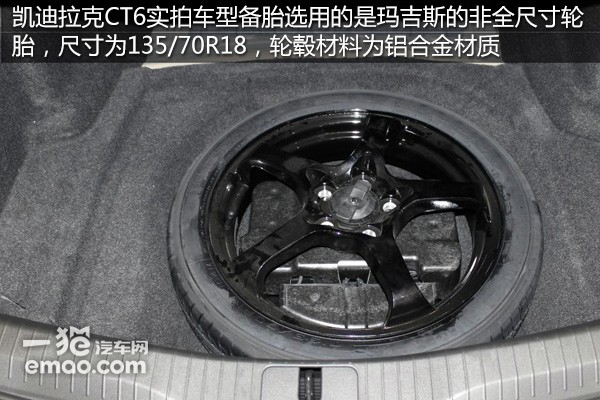编辑总结：作为凯迪拉克倾情打造的一款旗舰级别的豪华轿车，融合了凯迪拉克积累的多种黑科技，大胆前卫的外形设计，还是有很多购买它的理由，43.99万-81.88万的价格区间跨度，到底怎么选，还得看客官们的喜好和袋里的米了。（车辆提供：上海百联沪通汽车销售有限公司）

|分享

•已阅！握爪
•我手滑为你点赞
•128个赞！
•不明觉厉
•阅后既醉
•有钱！任性
•照片太美，我不敢看
•干货！杠杠的
•高大上
•膜拜中！
•请接受我的膝盖
•猫哥/猫妹么么哒
•神吐槽
•我只笑笑不说话
•我想静静
•窒息
##### 热度排行• 阿斯顿·马丁
• 奥迪
• 阿尔法罗密欧
• ALPINA
• 爱驰
• AC Schnitzer
• AITO
• ARCFOX极狐
• AUXUN傲旋

• 巴博斯
• 宝骏
• 宝马
• 保时捷
• 北汽制造
• 奔驰
• 奔腾
• 本田
• 比亚迪
• 标致
• 别克
• 宾利
• 布加迪
• 北汽威旺
• 北京
• 北汽绅宝
• 北汽幻速
• 北汽新能源
• 宝沃
• 比速汽车
• 北汽道达
• 比德文汽车
• 铂驰
• 北汽昌河
• 百智新能源
• 宝骐汽车
• 比克汽车
• 博速

• 昌河
• 长安
• 长城
• 长安商用
• 成功汽车
• 长江EV
• 长安轻车型
• 长安欧尚
• 长安凯程
• 车驰汽车
• 橙仕
• 创维汽车

• 大众
• 道奇
• 东风
• 东风风神
• 东风小康
• 东南
• DS
• 东风风行
• 东风风度
• 东风风光
• 电咖
• 大乘汽车
• 大运
• 东风富康
• 东风·瑞泰特
• 东风新能源
• 东风御风

• 法拉利
• 菲亚特
• 丰田
• 福迪
• 福特
• 福田汽车
• 福汽启腾
• 风诺
• 飞碟汽车
• 飞凡汽车

• GMC
• 广汽传祺
• 广汽吉奥
• 观致
• 国金汽车
• 国机智骏
• 高合汽车
• 广汽埃安

• 哈飞
• 海格
• 海马
• 华泰
• 黄海
• 恒天
• 红旗
• 哈弗
• 华颂
• 华凯
• 华泰新能源
• 汉腾汽车
• 华利
• 红星汽车
• HYCAN合创
• 汉龙汽车
• 恒润汽车
• 宏远汽车
• 华晨新日
• 华骐
• 华梓汽车

• Icona
• IMSA英飒

• Jeep
• 江淮
• 江铃
• 捷豹
• 金杯
• 九龙
• 吉利汽车
• 金旅
• 金龙
• 江铃集团轻汽
• 江铃集团新能源
• 君马汽车
• 捷途
• 钧天
• 捷达
• 几何汽车
• 极氪
• 捷尼赛思
• 金冠汽车

• 开瑞
• 凯迪拉克
• 科尼赛克
• 克莱斯勒
• KTM
• 卡威
• 凯翼
• 康迪
• 康迪电动汽车集团
• 开沃汽车
• 卡升
• 克蒂汽车
• 克慕勒

• 猎豹汽车
• 兰博基尼
• 劳斯莱斯
• 雷克萨斯
• 雷诺
• 理念
• 力帆
• 莲花汽车
• 林肯
• 铃木
• 陆风
• 路虎
• 路特斯
• 领志
• 领克
• 零跑汽车
• Lorinser
• LOCAL MOTORS
• 领途汽车
• 理想汽车
• 罗夫哈特
• 力帆汽车
• LEVC
• LIMGENE凌际
• LUMMA
• 莱茵汽车
• 岚图汽车
• 凌宝汽车
• 龙程汽车

• MG
• MINI
• 马自达
• 玛莎拉蒂
• 迈凯伦
• 摩根
• 迈莎锐
• 迈迈
• 摩登汽车

• 纳智捷
• 哪吒汽车

• 讴歌
• 欧宝
• 欧朗
• 欧拉
• 欧尚汽车

• 帕加尼
• Polestar极星
• 朋克汽车

• 奇瑞
• 启辰
• 起亚
• 前途
• 庆铃汽车
• 骐铃汽车
• 乔治·巴顿

• 日产
• 荣威
• 瑞麒汽车
• 如虎
• 瑞驰
• 瑞驰新能源
• 容大智造
• RAM
• 睿蓝汽车

### S

• 上汽大通
• smart
• 三菱
• 双环
• 双龙
• 斯巴鲁
• 斯柯达
• 萨博
• 思铭
• 赛麟
• SWM斯威汽车
• SRM鑫源
• 思皓
• SHELBY
• 上喆
• SERES赛力斯
• SONGSAN MOTORS
• 速达
• 申龙客车
• 神州

• TESLA
• 泰卡特
• 腾势
• 特斯拉
• 天际汽车
• TECHART
• 坦克

• 威麟
• 威兹曼
• 沃尔沃
• 五菱汽车
• 五十铃
• 潍柴英致
• WEY
• 蔚来
• 威马汽车
• WALD
• 瓦滋
• 伟昊汽车
• 魏牌

• 现代
• 雪佛兰
• 雪铁龙
• 西雅特
• 新特汽车
• 小鹏汽车
• 新宝骏
• 小虎
• 小猬汽车
• 晓奥汽车

• 野马汽车
• 一汽
• 依维柯
• 英菲尼迪
• 永源
• 驭胜
• 云度
• 宇通客车
• 云雀汽车
• 远程汽车
• 一汽凌河
• 雅升汽车
• 野马新能源
• 银隆新能源
• 御捷
• 裕路

• 中华
• 中兴
• 众泰
• 知豆
• 之诺
• 正道汽车
• 智点汽车
• 智己汽车
• 中国重汽VGV
• A
• B
• C
• D
• E
• F
• G
• H
• I
• J
• K
• L
• M
• N
• O
• P
• Q
• R
• S
• T
• U
• V
• W
• X
• Y
• Z

• 阿斯顿·马丁
• 奥迪
• 阿尔法罗密欧
• ALPINA
• 爱驰
• AC Schnitzer
• AITO
• ARCFOX极狐
• AUXUN傲旋

• 巴博斯
• 宝骏
• 宝马
• 保时捷
• 北汽制造
• 奔驰
• 奔腾
• 本田
• 比亚迪
• 标致
• 别克
• 宾利
• 布加迪
• 北汽威旺
• 北京
• 北汽绅宝
• 北汽幻速
• 北汽新能源
• 宝沃
• 比速汽车
• 北汽道达
• 比德文汽车
• 铂驰
• 北汽昌河
• 百智新能源
• 宝骐汽车
• 比克汽车
• 博速

• 昌河
• 长安
• 长城
• 长安商用
• 成功汽车
• 长江EV
• 长安轻车型
• 长安欧尚
• 长安凯程
• 车驰汽车
• 橙仕
• 创维汽车

• 大众
• 道奇
• 东风
• 东风风神
• 东风小康
• 东南
• DS
• 东风风行
• 东风风度
• 东风风光
• 电咖
• 大乘汽车
• 大运
• 东风富康
• 东风·瑞泰特
• 东风新能源
• 东风御风

• 法拉利
• 菲亚特
• 丰田
• 福迪
• 福特
• 福田汽车
• 福汽启腾
• 风诺
• 飞碟汽车
• 飞凡汽车

• GMC
• 广汽传祺
• 广汽吉奥
• 观致
• 国金汽车
• 国机智骏
• 高合汽车
• 广汽埃安

• 哈飞
• 海格
• 海马
• 华泰
• 黄海
• 恒天
• 红旗
• 哈弗
• 华颂
• 华凯
• 华泰新能源
• 汉腾汽车
• 华利
• 红星汽车
• HYCAN合创
• 汉龙汽车
• 恒润汽车
• 宏远汽车
• 华晨新日
• 华骐
• 华梓汽车

• Icona
• IMSA英飒

• Jeep
• 江淮
• 江铃
• 捷豹
• 金杯
• 九龙
• 吉利汽车
• 金旅
• 金龙
• 江铃集团轻汽
• 江铃集团新能源
• 君马汽车
• 捷途
• 钧天
• 捷达
• 几何汽车
• 极氪
• 捷尼赛思
• 金冠汽车

• 开瑞
• 凯迪拉克
• 科尼赛克
• 克莱斯勒
• KTM
• 卡威
• 凯翼
• 康迪
• 康迪电动汽车集团
• 开沃汽车
• 卡升
• 克蒂汽车
• 克慕勒

• 猎豹汽车
• 兰博基尼
• 劳斯莱斯
• 雷克萨斯
• 雷诺
• 理念
• 力帆
• 莲花汽车
• 林肯
• 铃木
• 陆风
• 路虎
• 路特斯
• 领志
• 领克
• 零跑汽车
• Lorinser
• LOCAL MOTORS
• 领途汽车
• 理想汽车
• 罗夫哈特
• 力帆汽车
• LEVC
• LIMGENE凌际
• LUMMA
• 莱茵汽车
• 岚图汽车
• 凌宝汽车
• 龙程汽车

• MG
• MINI
• 马自达
• 玛莎拉蒂
• 迈凯伦
• 摩根
• 迈莎锐
• 迈迈
• 摩登汽车

• 纳智捷
• 哪吒汽车

• 讴歌
• 欧宝
• 欧朗
• 欧拉
• 欧尚汽车

• 帕加尼
• Polestar极星
• 朋克汽车

• 奇瑞
• 启辰
• 起亚
• 前途
• 庆铃汽车
• 骐铃汽车
• 乔治·巴顿

• 日产
• 荣威
• 瑞麒汽车
• 如虎
• 瑞驰
• 瑞驰新能源
• 容大智造
• RAM
• 睿蓝汽车

### S

• 上汽大通
• smart
• 三菱
• 双环
• 双龙
• 斯巴鲁
• 斯柯达
• 萨博
• 思铭
• 赛麟
• SWM斯威汽车
• SRM鑫源
• 思皓
• SHELBY
• 上喆
• SERES赛力斯
• SONGSAN MOTORS
• 速达
• 申龙客车
• 神州

• TESLA
• 泰卡特
• 腾势
• 特斯拉
• 天际汽车
• TECHART
• 坦克

• 威麟
• 威兹曼
• 沃尔沃
• 五菱汽车
• 五十铃
• 潍柴英致
• WEY
• 蔚来
• 威马汽车
• WALD
• 瓦滋
• 伟昊汽车
• 魏牌

• 现代
• 雪佛兰
• 雪铁龙
• 西雅特
• 新特汽车
• 小鹏汽车
• 新宝骏
• 小虎
• 小猬汽车
• 晓奥汽车

• 野马汽车
• 一汽
• 依维柯
• 英菲尼迪
• 永源
• 驭胜
• 云度
• 宇通客车
• 云雀汽车
• 远程汽车
• 一汽凌河
• 雅升汽车
• 野马新能源
• 银隆新能源
• 御捷
• 裕路

• 中华
• 中兴
• 众泰
• 知豆
• 之诺
• 正道汽车
• 智点汽车
• 智己汽车
• 中国重汽VGV
• A
• B
• C
• D
• E
• F
• G
• H
• I
• J
• K
• L
• M
• N
• O
• P
• Q
• R
• S
• T
• U
• V
• W
• X
• Y
• Z

• 阿斯顿·马丁
• 奥迪
• 阿尔法罗密欧
• ALPINA
• 爱驰
• AC Schnitzer
• AITO
• ARCFOX极狐
• AUXUN傲旋

• 巴博斯
• 宝骏
• 宝马
• 保时捷
• 北汽制造
• 奔驰
• 奔腾
• 本田
• 比亚迪
• 标致
• 别克
• 宾利
• 布加迪
• 北汽威旺
• 北京
• 北汽绅宝
• 北汽幻速
• 北汽新能源
• 宝沃
• 比速汽车
• 北汽道达
• 比德文汽车
• 铂驰
• 北汽昌河
• 百智新能源
• 宝骐汽车
• 比克汽车
• 博速

• 昌河
• 长安
• 长城
• 长安商用
• 成功汽车
• 长江EV
• 长安轻车型
• 长安欧尚
• 长安凯程
• 车驰汽车
• 橙仕
• 创维汽车

• 大众
• 道奇
• 东风
• 东风风神
• 东风小康
• 东南
• DS
• 东风风行
• 东风风度
• 东风风光
• 电咖
• 大乘汽车
• 大运
• 东风富康
• 东风·瑞泰特
• 东风新能源
• 东风御风

• 法拉利
• 菲亚特
• 丰田
• 福迪
• 福特
• 福田汽车
• 福汽启腾
• 风诺
• 飞碟汽车
• 飞凡汽车

• GMC
• 广汽传祺
• 广汽吉奥
• 观致
• 国金汽车
• 国机智骏
• 高合汽车
• 广汽埃安

• 哈飞
• 海格
• 海马
• 华泰
• 黄海
• 恒天
• 红旗
• 哈弗
• 华颂
• 华凯
• 华泰新能源
• 汉腾汽车
• 华利
• 红星汽车
• HYCAN合创
• 汉龙汽车
• 恒润汽车
• 宏远汽车
• 华晨新日
• 华骐
• 华梓汽车

• Icona
• IMSA英飒

• Jeep
• 江淮
• 江铃
• 捷豹
• 金杯
• 九龙
• 吉利汽车
• 金旅
• 金龙
• 江铃集团轻汽
• 江铃集团新能源
• 君马汽车
• 捷途
• 钧天
• 捷达
• 几何汽车
• 极氪
• 捷尼赛思
• 金冠汽车

• 开瑞
• 凯迪拉克
• 科尼赛克
• 克莱斯勒
• KTM
• 卡威
• 凯翼
• 康迪
• 康迪电动汽车集团
• 开沃汽车
• 卡升
• 克蒂汽车
• 克慕勒

• 猎豹汽车
• 兰博基尼
• 劳斯莱斯
• 雷克萨斯
• 雷诺
• 理念
• 力帆
• 莲花汽车
• 林肯
• 铃木
• 陆风
• 路虎
• 路特斯
• 领志
• 领克
• 零跑汽车
• Lorinser
• LOCAL MOTORS
• 领途汽车
• 理想汽车
• 罗夫哈特
• 力帆汽车
• LEVC
• LIMGENE凌际
• LUMMA
• 莱茵汽车
• 岚图汽车
• 凌宝汽车
• 龙程汽车

• MG
• MINI
• 马自达
• 玛莎拉蒂
• 迈凯伦
• 摩根
• 迈莎锐
• 迈迈
• 摩登汽车

• 纳智捷
• 哪吒汽车

• 讴歌
• 欧宝
• 欧朗
• 欧拉
• 欧尚汽车

• 帕加尼
• Polestar极星
• 朋克汽车

• 奇瑞
• 启辰
• 起亚
• 前途
• 庆铃汽车
• 骐铃汽车
• 乔治·巴顿

• 日产
• 荣威
• 瑞麒汽车
• 如虎
• 瑞驰
• 瑞驰新能源
• 容大智造
• RAM
• 睿蓝汽车

### S

• 上汽大通
• smart
• 三菱
• 双环
• 双龙
• 斯巴鲁
• 斯柯达
• 萨博
• 思铭
• 赛麟
• SWM斯威汽车
• SRM鑫源
• 思皓
• SHELBY
• 上喆
• SERES赛力斯
• SONGSAN MOTORS
• 速达
• 申龙客车
• 神州

• TESLA
• 泰卡特
• 腾势
• 特斯拉
• 天际汽车
• TECHART
• 坦克

• 威麟
• 威兹曼
• 沃尔沃
• 五菱汽车
• 五十铃
• 潍柴英致
• WEY
• 蔚来
• 威马汽车
• WALD
• 瓦滋
• 伟昊汽车
• 魏牌

• 现代
• 雪佛兰
• 雪铁龙
• 西雅特
• 新特汽车
• 小鹏汽车
• 新宝骏
• 小虎
• 小猬汽车
• 晓奥汽车

• 野马汽车
• 一汽
• 依维柯
• 英菲尼迪
• 永源
• 驭胜
• 云度
• 宇通客车
• 云雀汽车
• 远程汽车
• 一汽凌河
• 雅升汽车
• 野马新能源
• 银隆新能源
• 御捷
• 裕路

• 中华
• 中兴
• 众泰
• 知豆
• 之诺
• 正道汽车
• 智点汽车
• 智己汽车
• 中国重汽VGV
• A
• B
• C
• D
• E
• F
• G
• H
• I
• J
• K
• L
• M
• N
• O
• P
• Q
• R
• S
• T
• U
• V
• W
• X
• Y
• Z